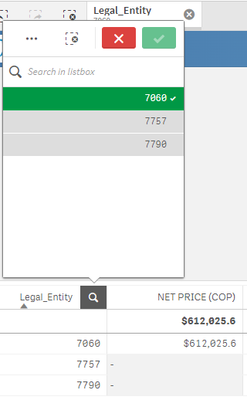Announcements
You can succeed best and quickest by helping others to succeed. Join the conversation.
cancel
Showing results for
Did you mean:Contributor II

## Filter is not working properly and brings null values for dimensions with no value

Hello all, currently I'm working on a table that brings the Sales Total by Legal Unit. I'm trying to display only the total of each legal unit, but when I filter it, it somehow brings me the three legal units, one with values and the other two with nulls. I used the disable null and also the not show zeroes, but it still shows the null values.

This is the code for the Measure

If(vCountry = 'LAN',
(Sum({<Year = {"\$(=Date(Max(Periodo), 'YYYY'))"}>}NTS_LAN))/(Sum({<Year = {"\$(=Date(Max(Periodo), 'YYYY'))"}>}Net_Units)),

If(vCountry = 'CO',
(Sum({<Year = {"\$(=Date(Max(Periodo), 'YYYY'))"}>}NTS))/(Sum({<Year = {"\$(=Date(Max(Periodo), 'YYYY'))"}>}Net_Units)),

If(vCountry = 'PEC',
(Sum({<Legal_Entity={'7790'},Year = {"\$(=Date(Max(Periodo), 'YYYY'))"}>}NTS) + Sum({<Legal_Entity={'7757'},Year = {"\$(=Date(Max(Periodo), 'YYYY'))"}>}NTS_LAN))/(Sum({<Year = {"\$(=Date(Max(Periodo), 'YYYY'))"}>}Net_Units)),

(Sum({<Year = {"\$(=Date(Max(Periodo), 'YYYY'))"}>}NTS))/(Sum({<Year = {"\$(=Date(Max(Periodo), 'YYYY'))"}>}Net_Units))
))
)

And this happens for all the other visualizations, including charts, what could be a possible solution?Labels (6)

• ### Variables